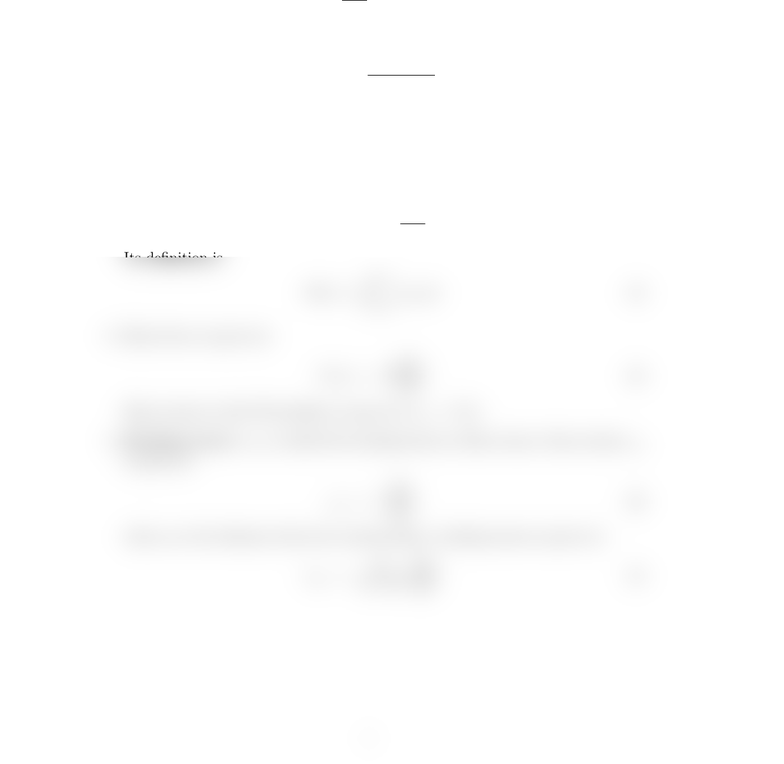Study Guides (400,000)
US (230,000)
UCSB (3,000)
EARTH (10)
Final

# EARTH 135 Lecture Notes - Lecture 6: Shear Stress, Shear ForceExam

Department
Earth Science
Course Code
EARTH 135
Professor
Tanimoto
Study Guide
Final

This preview shows half of the first page. to view the full 2 pages of the document.Earth 135 Lecture 6
Summary : Plate Bending
1. The basic equation
Dd4w
dx4=q(x) (1)
where Dis the ﬂexural ridigity given by
D=Eh3
12(1 ν2)(2)
with hbeing the thickness of a plate and (E, ν) are the Young’s modulus and
the Poisson’s ratio of a plate. Vertical displacement wis controlled by this
parameter.
2. Moment is given by
M(x) = Dd2w
dx2(3)
Its denition is
M(x) = Zh/2
h/2
zσxxdz (4)
3. Shear force is given by
V(x) = Dd3w
dx3(5)
Shear stress in this 2D problem is given by σxz =V/h.
4. Bending stress: σxx is called the bending stress or ber stress. Since strain ǫxx
is given by
ǫxx =zd2w
dx2(6)
where yis the distance from the neutral plane, bending stress is given by
σxx =E
1ν2zd2w
dx2(7)
1